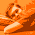## Thursday, July 28, 2011

### Fast 3D-vector matrix transformation using SSE4

In this blog-post we'd like to show how to efficiently transform multiple 3D vectors using an affine transformation matrix. Each vector has three coordinates (x, y and z) and the matrix consists of three rows each with 4 elements (3 for rotation/scale + 1 for translation). In order to multiply a 3-element vector with a 3x4 matrix, we add an additional 1 at the end of the 3D vector:

```| T00 T01 T02 T03 |   | x |   | x' |
| T10 T11 T12 T13 | * | y | = | y' |
| T20 T21 T22 T23 |   | z |   | z' |
| 1 |
```

Using SSE, the matrix-vector product can be performed straight forward. However, we want to process an array of `float3` elements and exploit 128-bit and 64-bit loads and stores. Therefore, we process two `float3` elements at the same time by using loop unrolling. At each iteration, we load the vectors, set the 4-th element to 1, matrix-multiply and store two results.

```static const __m128 MM_ONES = _mm_set1_ps(1.0f);

void multhom(float3* dst, const float4* matrix, const float3* src, int num) const
{

// unrolled loop
int i = 0;
for(; i < ROUND_DOWN(num, 2); i+=2)
{
__m128 xyzu = _mm_loadu_ps(&src[i].x);  // X0 Y0 Z0 X1
__m128 vw11 = _mm_loadl_pi(_mm_setzero_ps(), (const __m64*)&src[i+1].y); // Y1 Z1 1 1
// convert xyzuvw11 to xyz1 and uvw1
__m128 zu1v = _mm_shuffle_ps(xyzu, vw11, _MM_SHUFFLE(0, 2, 3, 2)); // Z0 X1 1 Y1
__m128 xyz1 = _mm_shuffle_ps(xyzu, zu1v, _MM_SHUFFLE(2, 0, 1, 0)); // X0 Y0 Z0 1
__m128 uvw1 = _mm_shuffle_ps(zu1v, vw11, _MM_SHUFFLE(3, 1, 3, 1)); // X1 Y1 Z1 1

// Perform matrix multiplication
__m128 x = _mm_mul_ps(xyz1, r0);
__m128 y = _mm_mul_ps(xyz1, r1);
__m128 z = _mm_mul_ps(xyz1, r2);

__m128 u = _mm_mul_ps(uvw1, r0);
__m128 v = _mm_mul_ps(uvw1, r1);
__m128 w = _mm_mul_ps(uvw1, r2);

// Prepare the 6 output values (x'y'z'u'v'w')
__m128 out_zuvw = _mm_shuffle_ps(uvzw, uvzw, _MM_SHUFFLE(3, 1, 0, 2));
__m128 out_xyzu = _mm_movelh_ps(xyzw, out_zuvw);

_mm_storeu_ps(&dst[i].x, out_xyzu);           // write X,Y,Z,U
_mm_storeh_pi((__m64*)&dst[i+1].y, out_zuvw); // write V,W
}
// tail for loop unrolling
if (i < num)
{
__m128 x = _mm_mul_ps(r0, v);
__m128 y = _mm_mul_ps(r1, v);
__m128 z = _mm_mul_ps(r2, v);
__m128 out = _mm_hadd_ps(xy, zw); // x,y,z,?
_mm_storel_pi((__m64*)&dst[i].x, out);
_mm_store_ss(&dst[i].z, _mm_movehl_ps(out, out));
}
}
```
This methods allows to transform an arbitrary number of `float3` vectors. The following example shows how to call it:
```float4 matrix = {/* fill the matrix */};
const float3* src = /* pointer to input values */;
float3* dst = /* pointer to output values */;

multhom(dst, matrix, src, numElements);
```

1.Very interesting. Can this be easily adapted to SSE3?

2.Yes, it is not so hard to do. The only SSE4 command is the _mm_insert_ps(), so just replace its functionality using SSE3 intrinsics. I rewrote the save-part of the unrolled loop using shuffle and unpacklo. The v-vector of the tail can be loaded using something like:
__m128 v = _mm_movelh_ps(xy00, z100);
which takes a few more cycles, but since it's called at most once, that should not cause any performance problem.

3.Note that the code using the _mm_loadl_pi/_mm_insert_ps statement for loading a homogeneous vector might not work in Release build with Visual C++ 2010 compiler. There is a bug in the compiler which I reported recently: https://connect.microsoft.com/VisualStudio/feedback/details/683152/wrong-release-code-generation-for-sse4-intrinsics

4.theowl84,
Now I stay in a state of shock:
fpu quicker than sse...

for(size_t i = 0; i < num; i++)
{
pDest[i].x = pSrc[i].x*pMatrix.x+pSrc*pMatrix[i].y.y+pSrc[i].z*pMatrix.z + pMatrix.u;
pDest[i].y = pSrc[i].x*pMatrix.x+pSrc[i].y*pMatrix.y+pSrc[i].z*pMatrix.z + pMatrix.u;
pDest[i].z = pSrc[i].x*pMatrix.x+pSrc[i].y*pMatrix.y+pSrc[i].z*pMatrix.z + pMatrix.u;}

nCicl = 1000 nPoints = 1000000
fpu time 15 sec
sse time 17 sec

1.This algorithm isn't exactly what I'd call "efficient," but there are several reasons this might happen:

1. You aren't compiling to use SSE intrinsics, and they're being compiled out into regular FPU instructions, with worse optimization.

2. You lose almost all the benefits of SSE if you're constantly loading and unloading the registers. You'll see a much better improvement once you perform more than a single translation before extracting the values into a float array.

3. A lot of these functions in this algorithm are being called as arguments to other functions, which tends to make it harder for the compiler to optimize (depending on which compiler you're using).

4. There's a LOT of horizontal addition going on here, which is one of the most expensive moves around. I would strongly suggest finding an alternative method of doing these steps, as they're quite costly, and can usually be avoided.